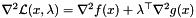Couenne  0.2
Couenne::ExprHess Class Reference

expression matrices. More...

#include <CouenneExprHess.hpp>

## Public Member Functions

ExprHess ()

ExprHess (CouenneProblem *)

ExprHess (const ExprHess &)

ExprHessoperator= (const ExprHess &)

ExprHessclone ()

~ExprHess ()

int nnz ()

int * iRow ()

int * jCol ()

int * numL ()

int ** lamI ()

expression *** expr ()

## Private Attributes

int nnz_
number of (symbolic) nonzeroes More...

int * iRow_
row indices (read this way by eval_h) More...

int * jCol_
col indices More...

int * numL_
There are m+1 (m constraints + 1 obj) components: More...

int ** lamI_
vector of indices in the lambda vector whose constraint has nonzero entry in this position of the hessian More...

expression *** expr_
list of lists of pointers to expression More...

## Detailed Description

expression matrices.

Used to evaluate the Hessian of the Lagrangian function at an optimal solution of the NLP

Definition at line 21 of file CouenneExprHess.hpp.

## Constructor & Destructor Documentation

 Couenne::ExprHess::ExprHess ( )
 Couenne::ExprHess::ExprHess ( CouenneProblem * )
 Couenne::ExprHess::ExprHess ( const ExprHess & )
 Couenne::ExprHess::~ExprHess ( )

## Member Function Documentation

 ExprHess& Couenne::ExprHess::operator= ( const ExprHess & )
 ExprHess* Couenne::ExprHess::clone ( )
 int Couenne::ExprHess::nnz ( )
inline

Definition at line 63 of file CouenneExprHess.hpp.

References nnz_.

 int* Couenne::ExprHess::iRow ( )
inline

Definition at line 64 of file CouenneExprHess.hpp.

References iRow_.

 int* Couenne::ExprHess::jCol ( )
inline

Definition at line 65 of file CouenneExprHess.hpp.

References jCol_.

 int* Couenne::ExprHess::numL ( )
inline

Definition at line 66 of file CouenneExprHess.hpp.

References numL_.

 int** Couenne::ExprHess::lamI ( )
inline

Definition at line 67 of file CouenneExprHess.hpp.

References lamI_.

 expression*** Couenne::ExprHess::expr ( )
inline

Definition at line 69 of file CouenneExprHess.hpp.

References expr_.

## Member Data Documentation

 int Couenne::ExprHess::nnz_
private

number of (symbolic) nonzeroes

Definition at line 25 of file CouenneExprHess.hpp.

Referenced by nnz().

 int* Couenne::ExprHess::iRow_
private

row indices (read this way by eval_h)

Definition at line 26 of file CouenneExprHess.hpp.

Referenced by iRow().

 int* Couenne::ExprHess::jCol_
private

col indices

Definition at line 27 of file CouenneExprHess.hpp.

Referenced by jCol().

 int* Couenne::ExprHess::numL_
private

There are m+1 (m constraints + 1 obj) components:Implementing a FP requires adding one for gg', the gradient again being set up at the beginning (at least its expression members are known).

This can simply be hacked by the FP itself. Same for the changed hessian, simply replace the CouenneProblem's objective with sum (objective, norm)

Actually, we could do the gg' trick by replacing the objective with sum (objective, norm, gg') size of each lamI_

Definition at line 44 of file CouenneExprHess.hpp.

Referenced by numL().

 int** Couenne::ExprHess::lamI_
private

vector of indices in the lambda vector whose constraint has nonzero entry in this position of the hessian

Definition at line 46 of file CouenneExprHess.hpp.

Referenced by lamI().

 expression*** Couenne::ExprHess::expr_
private

list of lists of pointers to expression

Definition at line 50 of file CouenneExprHess.hpp.

Referenced by expr().

The documentation for this class was generated from the following file: